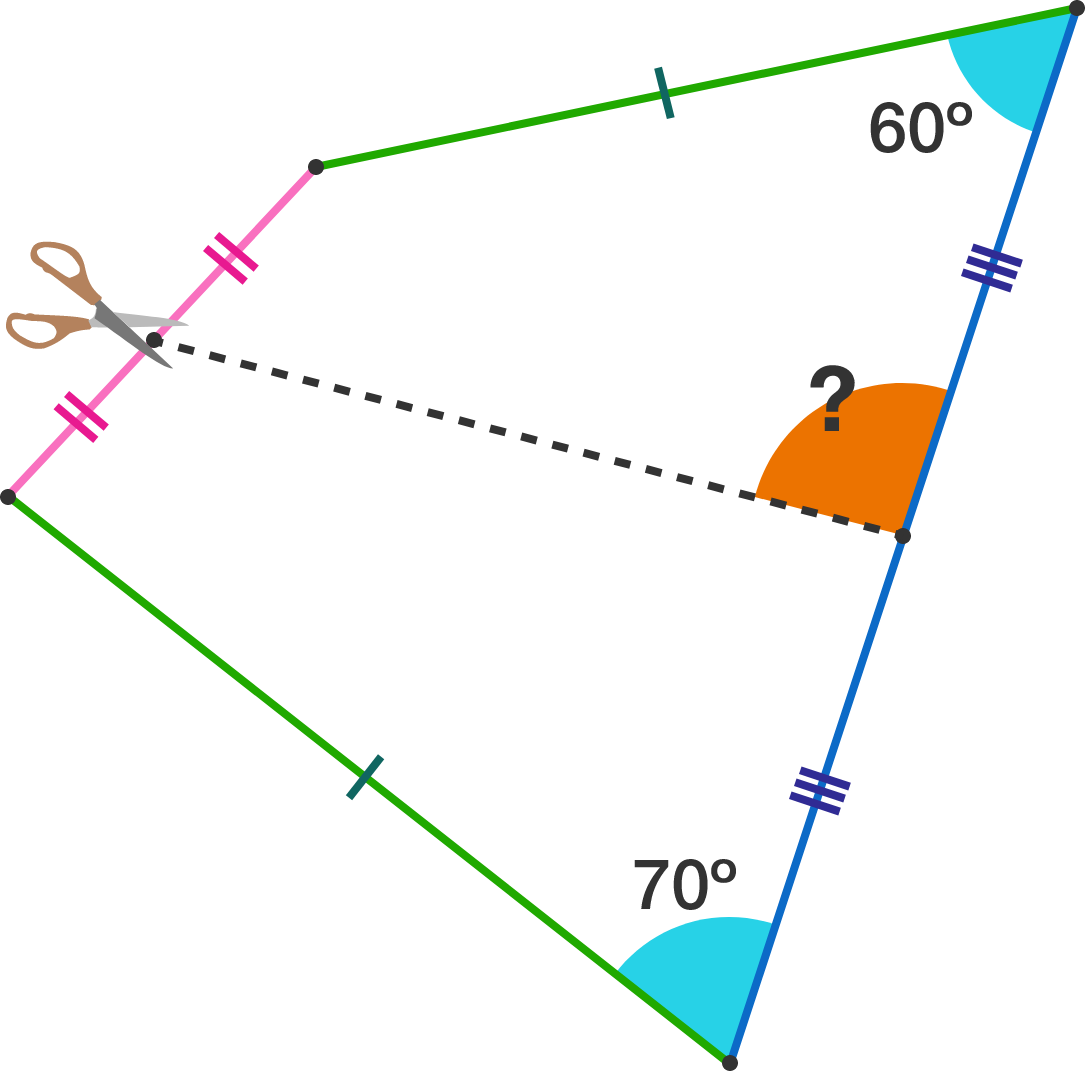# Find the value of this angle

Geometry Level 3

Samuel has decided to cut a quadrilateral into 2 parts such that the 2 resulting quadrilaterals have identical side lengths, as shown in the diagram. Given the 2 angles $60^\circ$ and $70^\circ,$ find the measure of the orange angle (in degrees).×